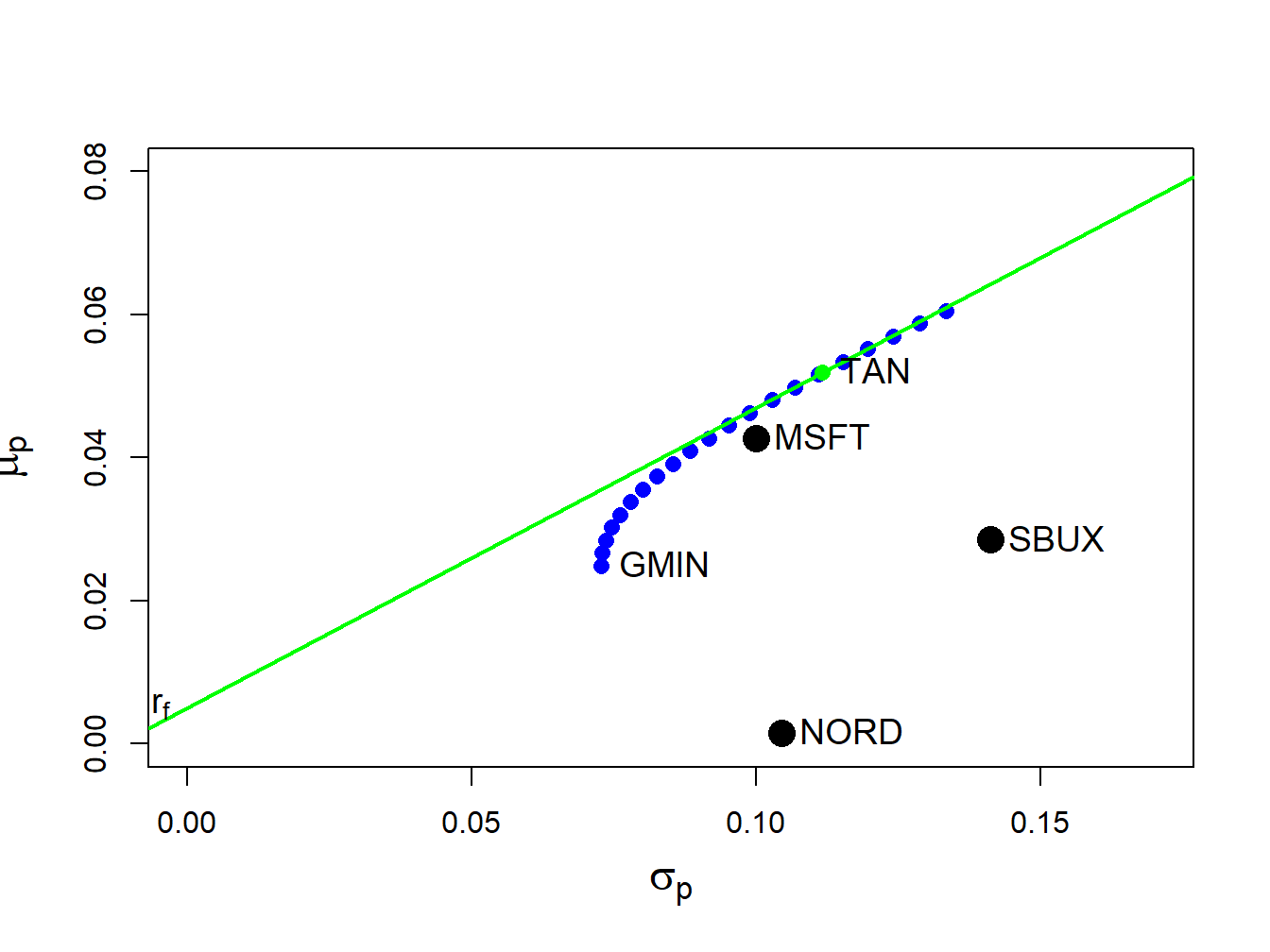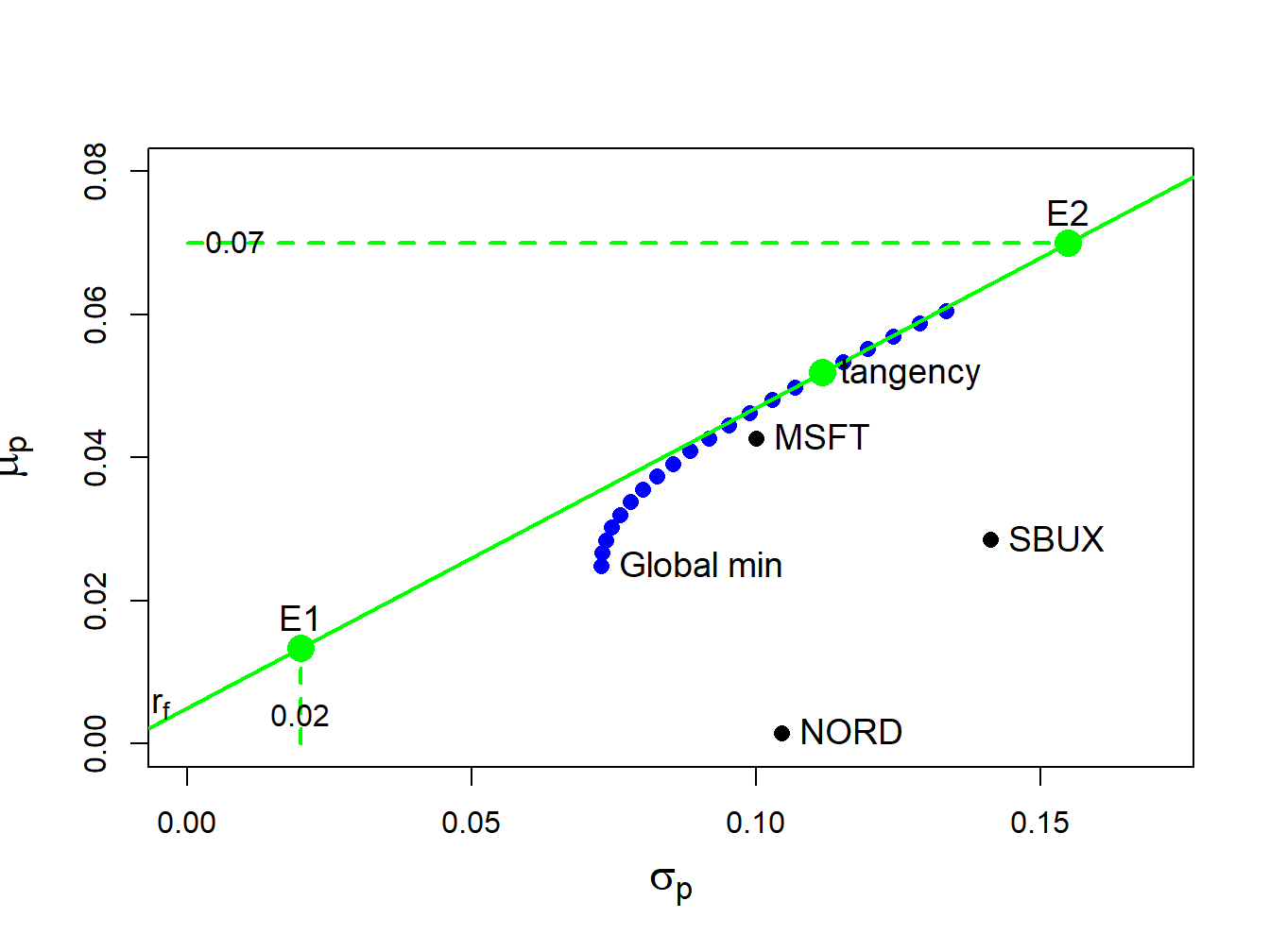## 12.5 Computing Efficient Portfolios of N risky Assets and a Risk-Free Asset Using Matrix Algebra

In Chapter 11, we showed that efficient portfolios of two risky assets and a single risk-free (T-Bill) asset are portfolios consisting of the highest Sharpe ratio portfolio (tangency portfolio) and the T-Bill. With three or more risky assets and a T-Bill the same result holds.

### 12.5.1 Computing the tangency portfolio using matrix algebra

The tangency portfolio is the portfolio of risky assets that has the highest Sharpe ratio. The tangency portfolio, denoted $$\mathbf{t}=(t_{\textrm{1}},\ldots,t_{N})^{\prime}$$, solves the constrained maximization problem: $\begin{equation} \underset{\mathbf{t}}{\max}~\frac{\mathbf{t}^{\prime}\mu-r_{f}}{(\mathbf{t}^{\prime}\Sigma \mathbf{t})^{{\frac{1}{2}}}}=\frac{\mu_{p,t}-r_{f}}{\sigma_{p,t}}\textrm{ s.t. }\mathbf{t}^{\prime}\mathbf{1}=1,\tag{12.25} \end{equation}$ where $$\mu_{p,t}=\mathbf{t}^{\prime}\mu$$ and $$\sigma_{p,t}=(\mathbf{t}^{\prime}\Sigma \mathbf{t})^{{\frac{1}{2}}}$$. The Lagrangian for this problem is: $L(\mathbf{t},\lambda)=\left(\mathbf{t}^{\prime}\mu-r_{f}\right)(\mathbf{t}^{\prime}\Sigma \mathbf{t})^{-{\frac{1}{2}}}+\lambda(\mathbf{t}^{\prime}\mathbf{1}-1).$ Using the chain rule, the first order conditions are: \begin{align*} \frac{\partial L(\mathbf{t},\lambda)}{\partial\mathbf{t}} & =\mu(\mathbf{t}^{\prime}\Sigma \mathbf{t})^{-{\frac{1}{2}}}-\left(\mathbf{t}^{\prime}\mu-r_{f}\right)(\mathbf{t}^{\prime}\Sigma \mathbf{t})^{-3/2}\Sigma \mathbf{t}+\lambda\mathbf{1}=\mathbf{0},\\ \frac{\partial L(\mathbf{t},\lambda)}{\partial\lambda} & =\mathbf{t}^{\prime}\mathbf{1}-1=0. \end{align*} After much tedious algebra, it can be shown that the solution for $$\mathbf{t}$$ has a nice simple expression: $\begin{equation} \mathbf{t}=\frac{\Sigma^{-1}(\mu-r_{f}\cdot\mathbf{1})}{\mathbf{1}^{\prime}\Sigma^{-1}(\mu-r_{f}\cdot\mathbf{1})}.\tag{12.26} \end{equation}$ The formula for the tangency portfolio (12.26) looks similar to the formula for the global minimum variance portfolio (12.8). Both formulas have $$\Sigma^{-1}$$ in the numerator and $$\mathbf{1}^{\prime}\Sigma^{-1}$$ in the denominator.

Remark

The location of the tangency portfolio, and the sign of the Sharpe ratio, depends on the relationship between the risk-free rate $$r_{f}$$ and the expected return on the global minimum variance portfolio $$\mu_{p,m}$$. If $$\mu_{p,m}>r_{f}$$, which is the usual case, then the tangency portfolio will have a positive Sharpe ratio. If $$\mu_{p,m}<r_{f}$$, which could occur when stock prices are falling and the economy is in a recession, then the tangency portfolio will have a negative Sharpe slope. In this case, efficient portfolios involve shorting the tangency portfolio and investing the proceeds in T-Bills.82

Example 3.6 (Computing the tangency portfolio)

Suppose $$r_{f}=0.005$$. To compute the tangency portfolio (12.26) in R for the three risky assets in Table 12.1 use:

##   MSFT   NORD   SBUX
##  1.027 -0.326  0.299

The tangency portfolio has weights $$t_{\textrm{msft}}=1.027,$$ $$t_{\textrm{nord}}=-0.326$$ and $$t_{\textrm{sbux}}=0.299,$$ and is given by the vector $$\mathbf{t}=(1.027,-0.326,0.299)^{\prime}.$$ Notice that Nordstrom, which has the lowest mean return, is sold short in the tangency portfolio. The expected return on the tangency portfolio, $$\mu_{p,t}=\mathbf{t}^{\prime}\mu$$, is:

mu.t = as.numeric(crossprod(t.vec, mu.vec))
mu.t
##  0.0519

The portfolio variance, $$\sigma_{p,t}^{2}=\mathbf{t}^{\prime}\Sigma \mathbf{t}$$, and standard deviation, $$\sigma_{p,t}$$, are:

sig2.t = as.numeric(t(t.vec)%*%sigma.mat%*%t.vec)
sig.t = sqrt(sig2.t)
c(sig2.t, sig.t)
##  0.0125 0.1116

Because $$r_{f}=0.005<\mu_{p,m}=0.0249$$ the tangency portfolio has a positive Sharpe’s ratio/slope given by:

SR.t = (mu.t - r.f)/sig.t
SR.t
##  0.42

The tangency portfolio is illustrated in Figure 12.9. It is the portfolio on the efficient frontier of risky assets in which a straight line drawn from the risk-free rate to the tangency portfolio (green line) is just tangent to the efficient frontier (blue dots).Figure 12.9: Tangency portfolio from example data.

$$\blacksquare$$

### 12.5.2 Alternative derivation of the tangency portfolio

The derivation of tangency portfolio formula (12.26) from the optimization problem (12.25) is a very tedious problem. It can be derived in a different way as follows. Consider forming portfolios of $$N$$ risky assets with return vector $$\mathbf{R}$$ and T-bills (risk-free asset) with constant return $$r_{f}$$. Let $$\mathbf{x}$$ denote the $$N\times1$$ vector of risky asset weights and let $$x_{f}$$ denote the safe asset weight and assume that $$\mathbf{x}^{\prime}\mathbf{1}+x_{f}=1$$ so that all wealth is allocated to these assets. The portfolio return is: $R_{p,x}=\mathbf{x}^{\prime}\mathbf{R}+x_{f}r_{f}=\mathbf{x}^{\prime}\mathbf{R}+(1-\mathbf{x}^{\prime}\mathbf{1})r_{f}=r_{f}+\mathbf{x}^{\prime}(\mathbf{R}-r_{f}\cdot\mathbf{1}).$ The portfolio excess return is: $\begin{equation} R_{p,x}-r_{f}=\mathbf{x}^{\prime}(\mathbf{R}-r_{f}\cdot\mathbf{1)}.\tag{12.27} \end{equation}$ The expected portfolio excess return (risk premium) and portfolio variance are: \begin{align} \mu_{p,x}-r_{f} & =\mathbf{x}^{\prime}(\mu-r_{f}\cdot\mathbf{1)},\tag{12.28}\\ \sigma_{p,x}^{2} & =\mathbf{x}^{\prime}\Sigma \mathbf{x}.\tag{11.5} \end{align} For notational simplicity, define $$\mathbf{\tilde{R}}=\mathbf{R}-r_{f}\cdot\mathbf{1}$$, $$\tilde{\mu}=\mu-r_{f}\cdot\mathbf{1}$$, $$\tilde{R}_{p,x}=R_{p,x}-r_{f}$$, and $$\tilde{\mu}_{p,x}=\mu_{p,x}-r_{f}$$. Then (12.27) and (12.28) can be re-expressed as: \begin{align} \tilde{R}_{p,x} & =\mathbf{x}^{\prime}\tilde{\mathbf{R}},\tag{12.29}\\ \tilde{\mu}_{p,x} & =\mathbf{x}^{\prime}\tilde{\mu}.\tag{12.30} \end{align} To find the minimum variance portfolio of risky assets and a risk free asset that achieves the target excess return $$\tilde{\mu}_{p,0}=\mu_{p,0}-r_{f}$$ we solve the minimization problem: $\min_{\mathbf{x}}~\sigma_{p,x}^{2}=\mathbf{x}^{\prime}\Sigma \mathbf{x}\textrm{ s.t. }\tilde{\mu}_{p,x}=\tilde{\mu}_{p,0}.$ Note that $$\mathbf{x}^{\prime}\mathbf{1}=1$$ is not a constraint because wealth need not all be allocated to the risky assets; some wealth may be held in the riskless asset. The Lagrangian is: $L(\mathbf{x},\lambda)=\mathbf{x}^{\prime}\mathbf{\Sigma x+}\lambda\mathbf{(x}^{\prime}\tilde{\mu}-\tilde{\mu}_{p,0}).$ The first order conditions for a minimum are: \begin{align} \frac{\partial L(\mathbf{x},\lambda)}{\partial\mathbf{x}} & =2\Sigma \mathbf{x}+\lambda\tilde{\mu}=0,\tag{12.31}\\ \frac{\partial L(\mathbf{x},\lambda)}{\partial\lambda} & =\mathbf{x}^{\prime}\tilde{\mu}-\tilde{\mu}_{p,0}=0.\tag{12.32} \end{align} Using the first equation (12.31), we can solve for $$\mathbf{x}$$ in terms of $$\lambda$$: $\begin{equation} \mathbf{x}=-\frac{1}{2}\lambda\Sigma^{-1}\tilde{\mu}.\tag{12.33} \end{equation}$ The second equation (12.32) implies that $$\mathbf{x}^{\prime}\tilde{\mu}=\tilde{\mu}^{\prime}\mathbf{x}=\tilde{\mu}_{p,0}$$. Then pre-multiplying (12.33) by $$\tilde{\mu}^{\prime}$$ gives: $\tilde{\mu}^{\prime}\mathbf{x=}-\frac{1}{2}\lambda\tilde{\mu}^{\prime}\Sigma^{-1}\tilde{\mu}=\tilde{\mu}_{p,0},$ which we can use to solve for $$\lambda$$: $\begin{equation} \lambda=-\frac{2\tilde{\mu}_{p,0}}{\tilde{\mu}^{\prime}\Sigma^{-1}\tilde{\mu}}.\tag{12.34} \end{equation}$ Plugging (12.34) into (12.33) then gives the solution for $$\mathbf{x}$$: $\begin{equation} \mathbf{x}=-\frac{1}{2}\lambda\Sigma^{-1}\tilde{\mu}=-\frac{1}{2}\left(-\frac{2\tilde{\mu}_{p,0}}{\tilde{\mu}^{\prime}\Sigma^{-1}\tilde{\mu}}\right)\Sigma^{-1}\tilde{\mu}=\tilde{\mu}_{p,0}\cdot\frac{\Sigma^{-1}\tilde{\mu}}{\tilde{\mu}^{\prime}\Sigma^{-1}\tilde{\mu}}.\tag{12.35} \end{equation}$ The solution for $$x_{f}$$ is then $$1-\mathbf{x}^{\prime}1$$.

Now, the tangency portfolio $$\mathbf{t}$$ is 100% invested in risky assets so that $$\mathbf{t}^{\prime}\mathbf{1}=\mathbf{1}^{\prime}\mathbf{t}=1$$. Using (12.35), the tangency portfolio satisfies: $\mathbf{1}^{\prime}\mathbf{t}=\tilde{\mu}_{p,t}\cdot\frac{\mathbf{1}^{\prime}\Sigma^{-1}\tilde{\mu}}{\tilde{\mu}^{\prime}\Sigma^{-1}\tilde{\mu}}=1,$ which implies that, $\begin{equation} \tilde{\mu}_{p,t}=\frac{\tilde{\mu}^{\prime}\Sigma^{-1}\tilde{\mu}}{\mathbf{1}^{\prime}\Sigma^{-1}\tilde{\mu}}.\tag{12.36} \end{equation}$ Plugging (12.36) back into (12.35) then gives an explicit solution for $$\mathbf{t}$$: \begin{align*} \mathbf{t} & \mathbf{=}\left(\frac{\tilde{\mu}^{\prime}\Sigma^{-1}\tilde{\mu}}{\mathbf{1}^{\prime}\Sigma^{-1}\tilde{\mu}}\right)\frac{\Sigma^{-1}\tilde{\mu}}{\tilde{\mu}^{\prime}\Sigma^{-1}\tilde{\mu}}=\frac{\Sigma^{-1}\tilde{\mu}}{\mathbf{1}^{\prime}\Sigma^{-1}\tilde{\mu}}\\ & =\frac{\Sigma^{-1}(\mu-r_{f}\cdot\mathbf{1})}{\mathbf{1}^{\prime}\Sigma^{-1}(\mu-r_{f}\cdot\mathbf{1})}, \end{align*} which is the result (12.26) we got from finding the portfolio of risky assets that has the maximum Sharpe ratio.

### 12.5.3 Mutual fund separation theorem again

When there is a risk-free asset (T-bill) available, the efficient frontier of T-bills and risky assets consists of portfolios of T-bills and the tangency portfolio. The expected return and standard deviation values of any such efficient portfolio are given by: \begin{align} \mu_{p}^{e} & =r_{f}+x_{t}(\mu_{p,t}-r_{f}),\tag{12.37}\\ \sigma_{p}^{e} & =x_{t}\sigma_{p,t},\tag{12.38} \end{align} where $$x_{t}$$ represents the fraction of wealth invested in the tangency portfolio ($$1-x_{t}$$ represents the fraction of wealth invested in T-Bills), and $$\mu_{p,t}=\mathbf{t}^{\prime}\mu$$ and $$\sigma_{p,t}=(\mathbf{t}^{\prime}\Sigma \mathbf{t})^{1/2}$$ are the expected return and standard deviation on the tangency portfolio, respectively. Recall, this result is known as the mutual fund separation theorem. The tangency portfolio can be considered as a mutual fund of the risky assets, where the shares of the assets in the mutual fund are determined by the tangency portfolio weights, and the T-bill can be considered as a mutual fund of risk-free assets. The expected return-risk trade-off of these portfolios is given by the line connecting the risk-free rate to the tangency point on the efficient frontier of risky asset only portfolios. Which combination of the tangency portfolio and the T-bill an investor will choose depends on the investor’s risk preferences. If the investor is very risk averse and prefers portfolios with very low volatility, then she will choose a combination with very little weight in the tangency portfolio and a lot of weight in the T-bill. This will produce a portfolio with an expected return close to the risk-free rate and a variance that is close to zero. If the investor can tolerate a large amount of volatility, then she will prefer a portfolio with a high expected return regardless of volatility. This portfolio may involve borrowing at the risk-free rate (leveraging) and investing the proceeds in the tangency portfolio to achieve a high expected return.

Example 2.25 (Efficient portfolios of three risky assets and T-bills chosen by risk averse and risk tolerant investors)

Consider the tangency portfolio computed from the example data in Table 12.1 with $$r_{f}=0.005$$. This portfolio is:

ans = c(t.vec, mu.t, sig.t)
names(ans)[4:5] = c("mu.t", "sigma.t")
ans
##    MSFT    NORD    SBUX    mu.t sigma.t
##  1.0268 -0.3263  0.2994  0.0519  0.1116

The efficient portfolios of T-Bills and the tangency portfolio is illustrated in Figure 12.10.

We want to compute an efficient portfolio that would be preferred by a highly risk averse investor, and a portfolio that would be preferred by a highly risk tolerant investor. A highly risk averse investor might have a low volatility (risk) target for his efficient portfolio. For example, suppose the volatility target is $$\sigma_{p}^{e}=0.02$$ or $$2\%$$. Using (12.38) and solving for $$x_{t}$$, the weights in the tangency portfolio and the T-Bill are:

x.t.02 = 0.02/sig.t
c(x.t.02, 1-x.t.02)
##  0.179 0.821

In this efficient portfolio, the weights in the risky assets are proportional to the weights in the tangency portfolio:

x.t.02*t.vec
##    MSFT    NORD    SBUX
##  0.1840 -0.0585  0.0537

The expected return and volatility values of this portfolio are:

mu.t.02 = x.t.02*mu.t + (1-x.t.02)*r.f
sig.t.02 = x.t.02*sig.t
c(mu.t.02, sig.t.02)
##  0.0134 0.0200

These values are illustrated in Figure 12.10 as the portfolio labeled “E1” .

A highly risk tolerant investor might have a high expected return target for his efficient portfolio. For example, suppose the expected return target is $$\mu_{p}^{e}=0.07$$ or $$7\%$$. Using (12.37) and solving for the $$x_{t}$$, the weights in the tangency portfolio and the T-Bill are:

x.t.07 = (0.07 - r.f)/(mu.t - r.f)
c(x.t.07, 1-x.t.07)
##   1.386 -0.386

Notice that this portfolio involves borrowing at the T-Bill rate (leveraging) and investing the proceeds in the tangency portfolio. In this efficient portfolio, the weights in the risky assets are:

x.t.07*t.vec
##   MSFT   NORD   SBUX
##  1.423 -0.452  0.415

The expected return and volatility values of this portfolio are:

mu.t.07 = x.t.07*mu.t + (1-x.t.07)*r.f
sig.t.07 = x.t.07*sig.t
c(mu.t.07, sig.t.07)
##  0.070 0.155

In order to achieve the target expected return of 7%, the investor must tolerate a 15.47% volatility. These values are illustrated in Figure 12.10 as the portfolio labeled “E2” .

$$\blacksquare$$Figure 12.10: Tangency portfolio

1. For a mathematical proof of these results, see Ingersoll (1987).↩︎